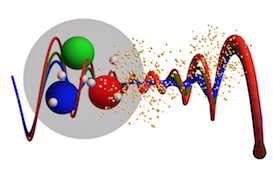#13th International workshop on Multiple Partonic Interactions at the LHC

14-18 November 2022

## Structure and production mechanism of $X(3872)$ in high-energy hadronic reactions.

17 Nov 2022, 12:25
18m
WG2: Double Parton Scattering

### Speaker

Antoni Szczurek (Institute of Nuclear Physics, PAN)

### Description

We calculate the total cross section and transverse momentum distributions
for the production of the enigmatic $\chi_{c1}(3872)$ (or X(3872))
assuming different scenarios:
$c \bar c$ state and $D^{0*} {\bar D}^0 + D^0 {\bar D}^{0*}$ molecule.
The derivative of the $c \bar c$ wave function needed in the first
scenario is taken from a potential model calculations.
Compared to earlier calculations of molecular state we include not only
single parton scattering (SPS) but also double parton scattering (DPS)
contributions.
The latter one seems to give smaller contribution than the SPS one.
The upper limit for the DPS production of
$\chi_{c1}(3872)$ is much below the CMS data.
We compare results of our calculations with existing experimental data
of CMS, ATLAS and LHCb collaborations.
Reasonable cross sections can be obtained in either $c \bar c$
or molecular $D {\bar D}^*$ scenarios for $X(3872)$, provided one takes
into account both directly produced $D^0, \bar D^0$, as well as
$D^0, \bar D^0$ from the decay of $D^*$. However arguments related
to the lifetime of $D^*$ suggest that the latter component may be not active.
With these reservations, also a hybrid scenario is not excluded.

The presentation will be based on:\
A. Cisek, W. Schafer and A. Szczurek, arXiv:2203.07827.

### Primary author

Antoni Szczurek (Institute of Nuclear Physics, PAN)

### Presentation Materials

###### Your browser is out of date!

Update your browser to view this website correctly. Update my browser now

×